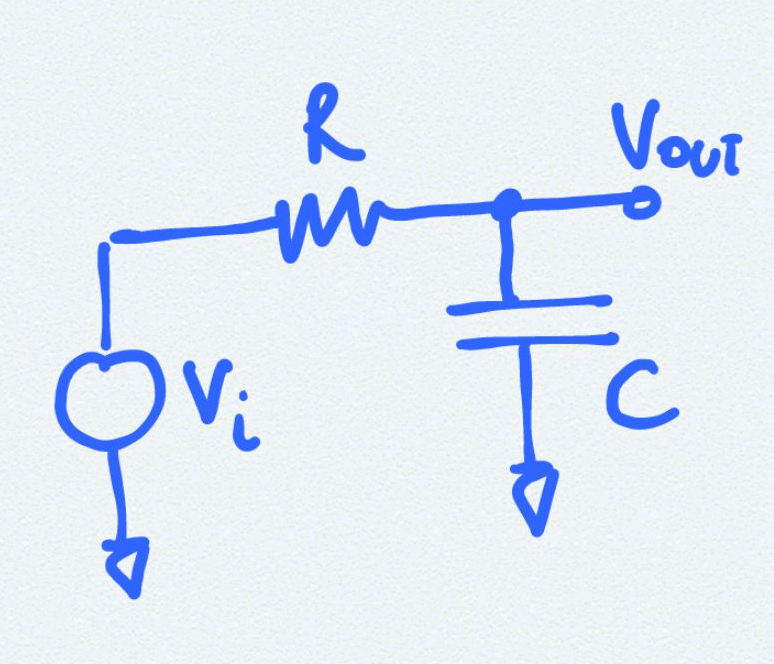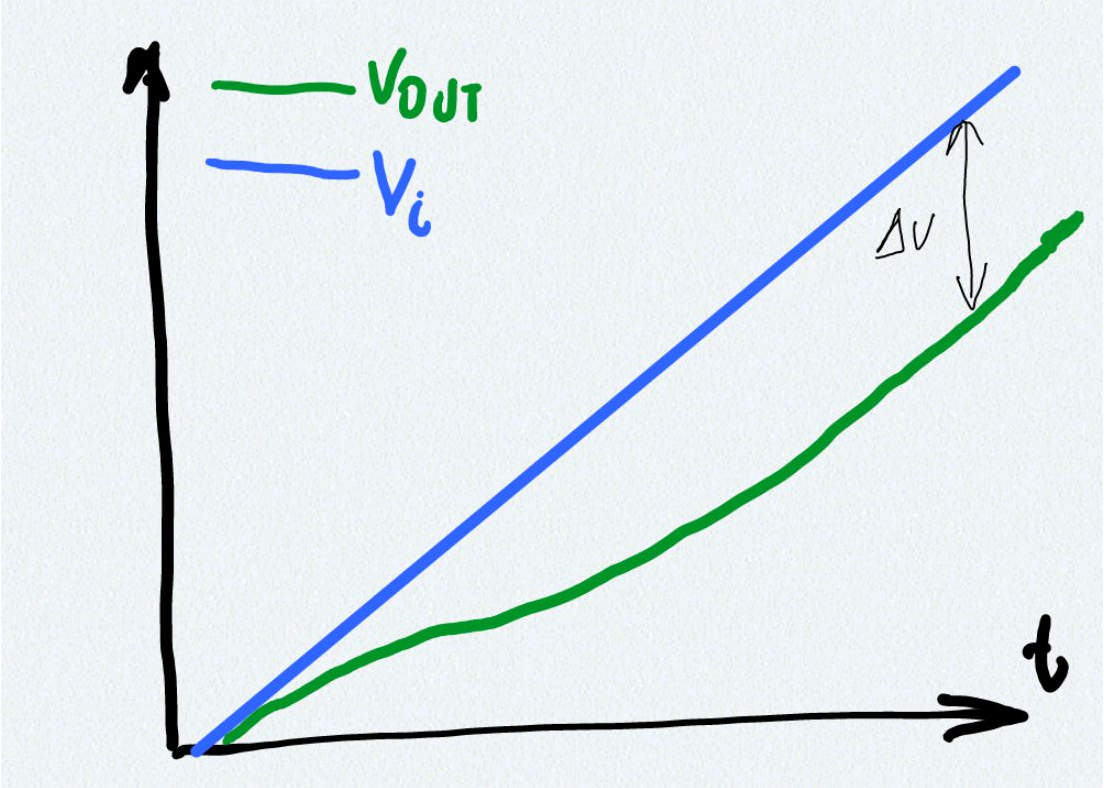# Maximum dV by dT a RC filter can follow# Circuit Diagram [top]# Analysis [top]

Although this is a very simple and well known circuit some interesting questions here and results here that we derive here is
• What happens when `\$V_i\$` is a ramp rather than a step.
• How does the output voltage behave different ramp slopesWhy are those important? If we know how soon the output catches up to a ramp and what is the voltage lag we can determine the RC values that give the desired response to a ramp input encountered so often in circuits.

Let `\$V_i=Mt\$` where M is the ramp slope and t is time so we have:
`\$\$V_i-V_{out}=RC{dV_{out} }/{dt}\$\$`

`\$\$Mt=RC{dV_{out} }/{dt}+V_{out}\$\$`

Solving this differential equation for `\$V_{out}\$` by multiplying with `\$e^{t/{RC}}\$` and integrating we get:
`\$\$V_{out}=Mt-MRC+A_oe^{-t/{RC}}\$\$`

where `\$A_o\$` is the integration constant which can be solved by the initial condition at t=0 `\$V_{out}=0\$`
Thus `\$A_o=MRC\$`
So we have:
`\$\$V_{out}=Mt+MRC(e^{-t/{RC}}-1)\$\$`

So now lets look at `\$V_{out}\$` after certain number of time constants say K i.e. `\$t=KRC\$` so we have:
`\$\$V_{out}=MKRC+MRC(e^{-K}-1)\$\$`

Here we can write `\$V_i=MKRC\$` therefore we have:
`\$\$V_i-V_{out}=MRC(1-e^{-K})\$\$`

Therefore the output would start tracking after a couple of time constants and the difference (voltage lag) between the output and the input is approximately MRC.

# Amplitude limited ramp [top]

An amplitude limited ramp is given as
`\$\$\table V_i, = ,Mt , t<t_F; ,=, Mt_F, t≥t_F\$\$`

To make a good ramp follower RC circuit the time `\$t_F≫RC\$` so that it starts following the ramp much before the ramp reaches its limit

| | | |
| | | |